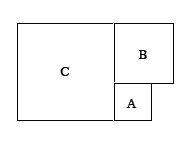### Home > A2C > Chapter 3 > Lesson 3.2.1 > Problem3-102

3-102.

In the diagram below, the area of square A is $121$ square units and the perimeter of square B is $80$ units. Find the area of square C.What does the given information tell you about the lengths of the sides of A and B?

Now that you know those lengths, what is the area of square C?